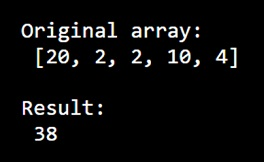# How to sum by year using NumPy?

Learn, how to sum by year using NumPy?
Submitted by Pranit Sharma, on December 30, 2022

NumPy is an abbreviated form of Numerical Python. It is used for different types of scientific operations in python. Numpy is a vast library in python which is used for almost every kind of scientific or mathematical operation. It is itself an array which is a collection of various methods and functions for processing the arrays.

## Sum by year using NumPy

Suppose we are given a NumPy array representing the data of some transactions of some years.

We need to aggregate the data of this array by the year values. For this purpose, we will use the numpy.sum() method.

Syntax:

```numpy.sum(
a,
axis=None,
dtype=None,
out=None,
keepdims=<no value>,
initial=<no value>,
where=<no value>
)
```

Parameter(s):

Some of the important parameters are,

• arr : input array.
• axis : the axis along which we want to calculate the sum value. Otherwise, it will consider arr to be flattened(works on all the axis). axis = 0 means along the column and axis = 1 means working along the row.
• out : Different array in which we want to place the result. The array must have the same dimensions as the expected output. The default is None.
• initial : [scalar, optional] Starting value of the sum.
• return : Sum of the array elements (a scalar value if the axis is none) or array with sum values along the specified axis.

Let us understand with the help of an example,

## Python code to sum by year using NumPy

```# Import numpy
import numpy as np

# Creating a numpy array
arr = [20, 2, 2, 10, 4]

# Display Original array
print("Original array:\n",arr,"\n")

# Using sum method
res = np.sum(arr)

# Display result
print("Result:\n",res)
```

Output: